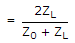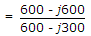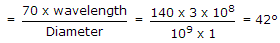# Electronics and Communication Engineering - Microwave Communication

### Exercise :: Microwave Communication - Section 2

16.

A transmission line has Z0 = 300 Ω and ZL = (300 - j300) ohm. The transmission coefficient is

 A. 1.265 ∠ - 18.43° B. 1.01 ∠ - 10° C. 1.14 ∠ 66.68° D. 1.09 ∠ 66.68°

Answer: Option A

Explanation:

Transmission coefficient= 1.265 ∠- 18.43°.

17.

In a reflex klystron oscillator, repeller electrode is at

 A. low positive potential B. high positive potential C. negative potential D. zero potential

Answer: Option C

Explanation:

It uses a single cavity resonator for generating microwave oscillations.

Its parts are electron gun, resonator, repeller and output coupling.

It operates on the principle of positive feed back.

The repeller electrode is at negative potential and sends the partially bunched electron beam back to resonator cavity.

This positive feedback supports oscillations. Its feature are:

1. Frequency range - 2 to 100 GHz

2. Power output - 10 MW to about 2 W

3. Efficiency - 10 - 20 %

Its applications include radar receivers, local oscillator in microwave devices, oscillator for microwave measurements in laboratories etc.

18.

A quarter wave line short circuited at load end behaves as

 A. an inductance B. an inductance and capacitance in series C. a capacitance D. an inductance and capacitance in parallel

Answer: Option D

Explanation:

A quarter wave line short-circuited at far end behaves as a parallel tuned circuit.

19.

A (75 - J50) ohm load is connected to a coaxial of Z0 = 75 ohm at 10 GHz. The best method of matching is to connect

 A. an inductance at load B. a short circuited stub at load C. a short circuited stub at specific distance from load D. a capacitance at specific distance from load

Answer: Option C

Explanation:

A short-circuited stub is a suitable method of matching.

20.

The width of a radio beam from a 1 m diameter parabolic antenna at 1 GHz is about

 A. 100° B. 50° C. 5° D. 2°

Answer: Option B

Explanation:

Beam width between nulls of a parabolic antenna. Therefore 50° is the nearest value.

#### Current Affairs 2021

Interview Questions and Answers